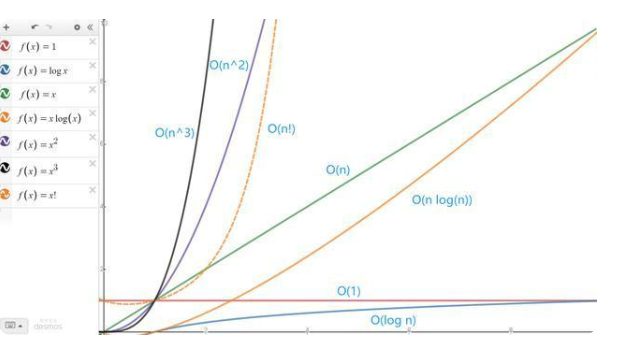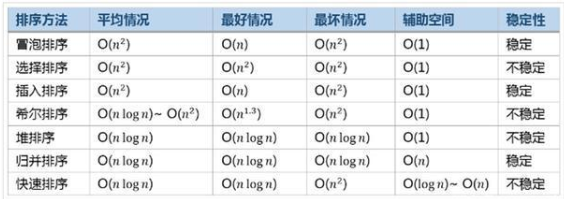# 时间复杂度和空间复杂度

9 篇文章 0 订阅

#### 一、时间复杂度

##### 2、原则
• 若运行时间是常数量级，则用常数 1 表示；
• 只保留时间函数中最高阶项
• 若最高阶项存在，则省去最高阶项前的系数；
##### 3、时间复杂度曲线O(1) > O(log2n)> o(n)> o(nlog2n) > o(n^2) > o(n^3) > o(2^n) > o(n!) > o(n^n)

##### 4、示例

（1） 常数阶 O(1)

public void test(n){
System.out.println(“1”);
System.out.println(“2”);
}



（2）线性阶 O(n)

计算1+2+3+....+n的和

int sum=0
for(i=0;i<n;i++){
sum+=i
}



（3）平(立)方阶

int sum=0;
for(int i=0;i<n;i++){
for(int j=0;j<n;j++){
sum+=j
}
}



（4）特殊平方阶

例如：O(n^2/2+n/2)->O(n^2)

for(){
for(){
.....            ----------->n^2
}
}
+
for(){
.....             ------------> n
}
System.out.println(“1”);



（5）对数阶

例如：O(log2n)

while(n>=1){
n=n/2;
}

n    执行次数
1      1
2      2
3      2

n          n/2        n/2/2      n/2/2/2    n/2/2/...
20--------->10---------->5-------->2.5------->1

n*（1/(2^m)）=1;



1、a^(log(a)(b))=b   =>log(a)(1)=0,log(a)(a)=1
2、log(a)(MN)=log(a)(M)+log(a)(N);
3、log(a)(M÷N)=log(a)(M)-log(a)(N);
4、log(a)(M^n)=nlog(a)(M)
5、log(a^n)M=1/nlog(a)(M)

##### 5、常见排序算法的时间复杂度情况#### 二、空间复杂度

##### 2、定义

• 如果程序所占用的存储空间和输入值无关，则该程序的空间复杂度就为 O(1)；反之，如果有关，则需要进一步判断它们之间的-关系：
• 如果随着输入值 n 的增大，程序申请的临时空间成线性增长，则程序的空间复杂度用 O(n) 表示;
• 如果随着输入值 n 的增大，程序申请的临时空间成 n2 关系增长，则程序的空间复杂度用 O(n2) 表示；
• 如果随着输入值 n 的增大，程序申请的临时空间成 n3 关系增长，则程序的空间复杂度用 O(n3) 表示；
06-252万+
09-2028万+08-013515
08-268330
02-0912万+
08-143万+
11-08972
07-153万+
12-021万+
08-27610
07-133008
09-161万+
09-0410万+
11-221万+点击重新获取扫码支付余额充值